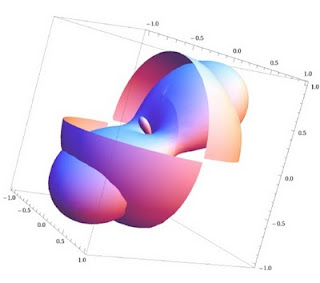## 20 September, 2011

### Mathematica and Spherical Harmonics

As my previous post about Mathematica seemed to be well-received, I've decided to dig some old code, add some comments and post it here. Unfortunately it's littered with \[symbol] tags as in Mathematica I used some symbols for variables and shortcuts (which you can enter either in that form or as esc-symbol-esc). You can also see a PDF version of the notebook here, with proper formatting. Enjoy!A function and its SH approximation
(* Normalization part of spherical harmonics *)
shNormalizationCoeffs[l_, m_] := Sqrt[((2*l + 1)*(l - m)!)/(4*Pi*(l + m)!)]

(* Evaluates to a function of \[Theta],\[Phi] for a given degree l and order m, it's defined as three different cases for m=0, m<0, and m>0*)
shGetFn[l_, m_] := Simplify[Piecewise[{{shNormalizationCoeffs[l, 0]*LegendreP[l, 0, Cos[\[Theta]]], m == 0}, {Sqrt*shNormalizationCoeffs[l, m]*Cos[m*\[Phi]]*LegendreP[l, m, Cos[\[Theta]]], m > 0}, {Sqrt*shNormalizationCoeffs[l, -m]*Sin[-m*\[Phi]]*LegendreP[l, -m, Cos[\[Theta]]], m < 0}}]]

(* Indices for a SH of a given degree, applies a function which creates a range from -x to x to every element of the range 0...l, return a list of lists. Note that body& is one of Mathematica's way to express pure function, with parameters #1,#2... fn/@list is the shorthand for Map[fn,list] *)
shIndices[l_] := (Range[-#1, #1] &) /@ Range[0, l]

(* For each element of the shIndices list, it replaces the corresponding shGetFn *)
(* This is tricky. MapIndexed takes a function of two parameters: element of the list and index in the list. Our function is itself a function applied to a list, as our elements are lists (shIndices is a list of lists) *)
shFunctions[l_] := MapIndexed[{list, currLevel} \[Function] ((m \[Function] shGetFn[currLevel - 1, m]) /@ list), shIndices[l]]

(* Generates SH coefficients of a given function fn of \[Theta],\[Phi], it needs a list of SH bases obtained from shFunctions,it will perform spherical integration between fn and each of the SH functions *)
shGenCoeffs[shfns_, fn_] := Map[Integrate[#1*fn[\[Theta], \[Phi]]*Sin[\[Theta]], {\[Theta], 0, Pi}, {\[Phi], 0, 2*Pi}] &, shfns]

(* From SH coefficients and shFunctions it will generate a function of \[Theta],\[Phi] which is the SH representation of the given coefficients. Note the use of assumptions over \[Theta] and \[Phi] passed as options to Simplify to be able to reduce the function correctly, @@ is the shorthand of Apply[fn,params] *)
angleVarsDomain = {Element[\[Theta], Reals], Element[\[Phi], Reals], \[Theta] >= 0, \[Phi] >= 0, \[Theta] <=
Pi, \[Phi] >= 2*Pi};
shReconstruct[shfns_, shcoeffs_] := Simplify[Plus @@ (Flatten[shcoeffs]*Flatten[shfns]), Assumptions -> angleVarsDomain]

(* Let's test what we have so far *)
testNumLevels = 2;
shfns = shFunctions[testNumLevels]
testFn[\[Theta]_, \[Phi]_] := Cos[\[Theta]]^10*UnitStep[Cos[\[Theta]]] (* Simple, symmetric around the z-axis *)

(* generate coefficients and reconstructed SH function *)
testFnCoeffs = shGenCoeffs[shfns, testFn]
testFnSH = {\[Theta], \[Phi]} \[Function]Evaluate[shReconstruct[shfns, testFnCoeffs]]

(* plot original and reconstruction *)
SphericalPlot3D[{testFn[\[Theta], \[Phi]], testFnSH[\[Theta], \[Phi]]}, {\[Theta], 0,
Pi}, {\[Phi], 0, 2*Pi}, Mesh -> False, PlotRange -> Full]

(* Checks if a given set of coefficients corresponds to zonal harmonics *)
shIsZonal[shcoeffs_, l_] := Plus @@ (Flaten[shIndices[l]]*Flatten[shcoeffs]) == 0

(* Some utility functions *)
shSymConvolveNormCoeffs[l_] := MapIndexed[{list, currLevel} \[Function] Table[Sqrt[4*Pi/(2*currLevel + 1)], {Length[list]}], shIndices[l]]
shExtractSymCoeffs[shcoeffs_] := Table[#1[[Ceiling[Length[#1]/2]]], {Length[#1]}] & /@ shcoeffs

(* Convolution with a kernel expressed via zonal harmonics, symmetric around the z-axis *)
shSymConvolution[shcoeffs_, shsymkerncoeffs_, l_] := (Check[shIsZonal[shsymkerncoeffs], err];
shSymConvolveNormCoeffs[l]*shcoeffs*shExtractSymCoeffs[shsymkerncoeffs])

(* Another test *)
testFn2[\[Theta]_, \[Phi]_] := UnitStep[Cos[\[Theta]]*Sin[\[Phi]]] (* asymmetric *)
testFn2Coeffs = shGenCoeffs[shfns, testFn2]
testFn2SH = {\[Theta], \[Phi]} \[Function]Evaluate[shReconstruct[shfns, testFn2Coeffs]]
plotFn2 = SphericalPlot3D[testFn2[\[Theta], \[Phi]], {\[Theta], 0, Pi}, {\[Phi], 0, 2*Pi}, Mesh -> False, PlotRange -> Full]
plotFn2SH = SphericalPlot3D[testFn2SH[\[Theta], \[Phi]], {\[Theta], 0, Pi}, {\[Phi], 0, 2*Pi}, Mesh -> False, PlotRange -> Full]
Show[plotFn2, plotFn2SH]

(* Test convolution *)
shIsZonal[testFnCoeffs, testNumLevels]
testConvolvedCoeffs = shSymConvolution[testFn2Coeffs, testFnCoeffs, testNumLevels]
testFnConvolvedSH = {\[Theta], \[Phi]} \[Function] Evaluate[shReconstruct[shfns, testConvolvedCoeffs]]
plotConvolvedSH = SphericalPlot3D[testFnConvolvedSH[\[Theta], \[Phi]], {\[Theta], 0, Pi}, {\[Phi], 0, 2*Pi}, Mesh -> False, PlotRange -> Full]